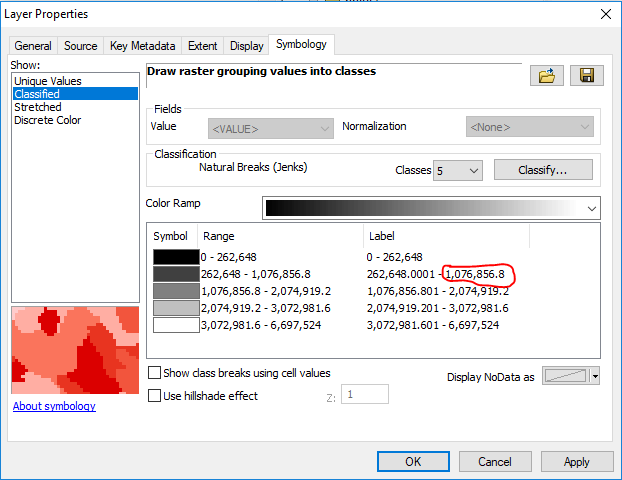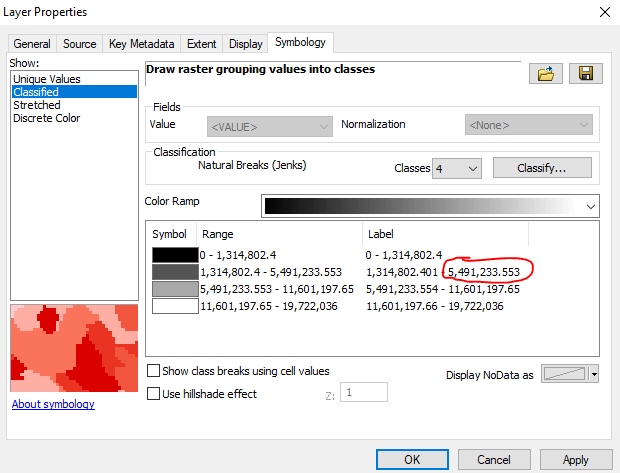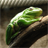# How do you extract Classified values (Break Values) from a Raster?

1536
4
08-04-2017 12:20 PMNew Contributor III

Hey I am trying to write a python code and I want to use Break value number as a condition in a loop. I am planning on using many different rasters for this program, so I want the program to accomadate any break values.in this example I would like to use 1,076,856.8 as my condition number.in this example I would like to use 5,491,233.553 as my condition number.

I understand that every raster will have different default classes depending on how the zonal histogram calculator works. But I would like for it to remain consistent for every Raster I use. What would be the best way to get this number while using the Python language?

4 RepliesOccasional Contributor II

The RasterClassifiedSymbology—Help | ArcGIS for Desktop arcpy.mapping class is probably what you are looking for.New Contributor III

If I were trying to read the break values. How would I do it? The code below would change the break values. Also I would I use raster in this example?

import arcpy
mxd = arcpy.mapping.MapDocument("CURRENT")
df = arcpy.mapping.ListDataFrames(mxd, "Lakes")
lyr = arcpy.mapping.ListLayers(mxd, "lakene.tif", df)
if lyr.symbologyType == "RASTER_CLASSIFIED":
lyr.symbology.classBreakValues = [1, 60, 118, 165, 255]
lyr.symbology.classBreakLabels = ["1 to 60", "61 to 118",
"119 to 165", "166 to 255"]
lyr.symbology.classBreakDescriptions = ["Class A", "Class B",
"Class C", "Class D"]
lyr.symbology.excludedValues = '0'
arcpy.RefreshActiveView()
arcpy.RefreshTOC()Frequent Contributor

The class break values returns a list, so it would be fairly easy to set the break values to a variable and then access them via a loop or use index values.

``````import arcpy
mxd = arcpy.mapping.MapDocument("CURRENT")
df = arcpy.mapping.ListDataFrames(mxd, "Lakes")
lyr = arcpy.mapping.ListLayers(mxd, "lakene.tif", df)
if lyr.symbologyType == "RASTER_CLASSIFIED":
values = lyr.symbology.classBreakValues
for val in values:
print val #checking value
#do something else with the values here.
‍‍‍‍‍‍‍‍‍‍``````

You could also just read them to a variable and then do something with them outside of the loop that you had set-up there, the important thing is to reading them into a variable so you have access to them for later in your script.  As the help file says the values for classBreakValues can be read or written, so you can check what they currently are and also have the ability to write new values to the layer symbology in the example you posted.  You want to just read what they currently are.

I think the better question is how are you going to set the class break values for every raster prior to doing the processing you want.  A raster file by itself has no symbology at all, only the values stored in it.  A layer file either in a map document or on a hard disk would have to be created for you to actually have break values showing the different symbolization of the raster values.  Your script would have to work with layer files that you have already created, or you can have a single layer file set-up with the symbology and break types and numbers you are looking for and apply them to a raster layer created from each raster and then creating the break values using the reclassify() method seen in the help.

Are you planning to use the same symbology method and number of classes each time, or will it vary raster to raster?  How many raster layer break values are you needing to make and read?New Contributor III

I am using model builder and python to delineate all the major watersheds on a dem. I am using model builder to create the flow direction and flow accumulation. I am using a python script to delineate all the major watersheds. For the python script I have a loop that needs to end on a condition number and I would like that condition number to be one of the break values because if I have the condition number set to 0 it will start making watersheds that are really small and unimportant for my use. So I am going to remain consistent and use that code you provided for me and use the second value in the loop. I do have a problem using it in different documents. It works in one document but it does not work in another document. The document that does not work the code runs but it does not read the break values in the layer and the layer is already created and has break values. So I am not sure what is wrong with it. What would be the best way to fix this issue?

import arcpy
from arcpy.sa import *
from arcpy import env

Flowaccumulation=r"C:\Temp\ARCGIS_PROJECT\Tutorial\new\fa"
flowdirection= r"C:\Temp\ARCGIS_PROJECT\Tutorial\new\fd"
pointcount=50

mxd=arcpy.mapping.MapDocument(r"CURRENT")
df=arcpy.mapping.ListDataFrames(mxd)
lyr=arcpy.mapping.ListLayers(mxd,"fa",df)
count=1
if lyr.symbologyType == "RASTER_CLASSIFIED":
values= lyr.symbology.classBreakValues
for val in values:
if count==2:
Condition=val
break
count= count + 1
maxValue= Condition + 1

while maxValue > Condition: